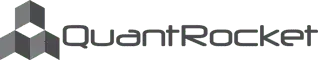Disclaimer

# Moonshot Strategy¶

This notebook introduces the Moonshot calendar spread trading strategy code and runs an example backtest.

## Moonshot strategy code¶

### Code highlights¶

In prices_to_signals, we use the function get_contract_nums_reindexed_like to isolate the bids and asks of the contract months from which we wish to create the calendar spread:

CONTRACT_NUMS = (1,2)
...

# Get a DataFrame of contract numbers and a Boolean mask of the
# contract nums constituting the spread
contract_nums = get_contract_nums_reindexed_like(bids, limit=max(self.CONTRACT_NUMS))
are_month_a_contracts = contract_nums == self.CONTRACT_NUMS
are_month_b_contracts = contract_nums == self.CONTRACT_NUMS

# Get a Series of bids and asks for the respective contract months by
# masking with contract num and taking the mean of each row (relying on
# the fact that the mask leaves only one observation per row)
month_a_bids = bids.where(are_month_a_contracts).mean(axis=1)

month_b_bids = bids.where(are_month_b_contracts).mean(axis=1)


To reflect the fact that we must buy at the ask and sell at the bid, we compute the spread differently for the purpose of identifying long vs short opportunities:

# Buying the spread means buying the month A contract at the ask and
# selling the month B contract at the bid

...

# Selling the spread means selling the month A contract at the bid


In positions_to_gross_returns, we model buying at the ask and selling at the bid:

are_buys = positions.diff() > 0
are_sells = positions.diff() < 0
midpoints = (bids + asks) / 2
bids.where(are_sells)).fillna(midpoints)



### Install strategy file¶

Install the strategy by moving it to the /codeload/moonshot directory:

## Example Backtest¶

The moonshot file contains an example subclass for backtesting the strategy with CL contract months 1 and 2:

class CLCalendarSpreadStrategy(CalendarSpreadStrategy):

UNIVERSES = "cl-fut"
DB = "cl-1min-bbo"
CONTRACT_NUMS = (1, 2)
BBAND_LOOKBACK_WINDOW = 60
BBAND_STD = 2
COMMISSION_CLASS = NymexCommission


There is also a "frictionless" version that ignores commissions and assumes fills at the bid-ask midpoint.

class FrictionlessCLCalendarSpreadStrategy(CLCalendarSpreadStrategy):


We run the backtest in monthly segments (segment="M"), which mirrors the sharding logic of our database.